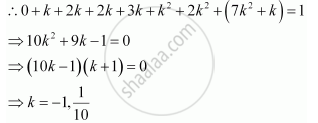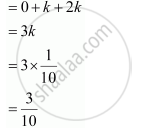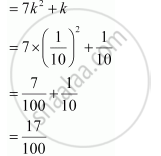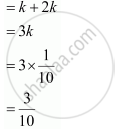Share

# A Random Variable X Has the Following Probability Distribution. Determine K, P (X < 3), P (X > 6), P (0 < X < 3) - CBSE (Science) Class 12 - Mathematics

ConceptRandom Variables and Its Probability Distributions

#### Question

A random variable X has the following probability distribution.

 X 0 1 2 3 4 5 6 7 P(X) 0 k 2k 2k 3k k2 2k2 7k2 + k

Determine

(i) k

(ii) P (X < 3)

(iii) P (X > 6)

(iv) P (0 < X < 3)

#### Solution

(i) It is known that the sum of probabilities of a probability distribution of random variables is one.k = − 1 is not possible as the probability of an event is never negative.

:.k = 1/10

(ii) P (X < 3) = P (X = 0) + P (X = 1) + P (X = 2)(iii) P (X > 6) = P (X = 7)(iv) P (0 < X < 3) = P (X = 1) + P (X = 2)Is there an error in this question or solution?

#### Video TutorialsVIEW ALL 

Solution A Random Variable X Has the Following Probability Distribution. Determine K, P (X < 3), P (X > 6), P (0 < X < 3) Concept: Random Variables and Its Probability Distributions.
S